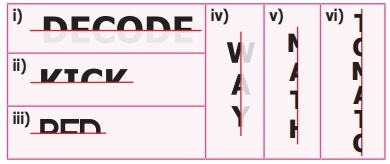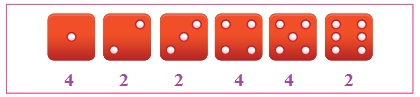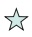Home | | Maths 6th Std | Exercise 4.1

# Exercise 4.1

6th Maths : Term 3 Unit 4 : Symmetry : Exercise 4.1 : Text Book Back Exercises Questions with Answers, Solution

Exercise 4.1

1. Fill in the blanks

i) The reflected image of the letter ‘q’ is P.

ii) A rhombus has two lines of symmetry.

iii) The order of rotational symmetry of the letter ‘z’ is two.

iv) A figure is said to have rotational symmetry, if the order of rotation is atleast two.

v) Translation symmetry occurs when an object slides to new position.

2. Say True or False

i) A rectangle has four lines of symmetry. [False ]

ii) A shape has reflection symmetry if it has a line of symmetry. [True]

iii) The reflection of the name RANI is[False ]

iv) Order of rotation of a circle is infinite. [True]

v) The number |9| has rotational symmetry. [False ]

3. Match the following shapes with their number of lines of symmetry.

i) Square a) No line of symmetry

ii) Parallelogram b) One line of symmetry

iii) Isosceles triangle c) Two lines of symmetry

iv) Rectangle d) Four lines of symmetry

i) Square d) Four lines of symmetry

ii) Parallelogram a) No line of symmetry

iii) Isosceles triangle b) One line of symmetry

iv) Rectangle c) Two lines of symmetry

4. Draw the lines of symmetry of the following.(i) 4 symmetry lines

(ii) 3 symmetry lines

(iii) 4 symmetry lines

(iv) 2 symmetry lines

5. Using the given horizontal line / vertical line as a line of symmetry, complete each alphabet to discover the hidden word.i) DECODE

ii) KICK

iii) BED6. Draw a line of symmetry of the given figures such that one hole coincide with the other hole(s) to make pairs.7. Complete the other half of the following figures such that the dotted line is the line of symmetry.8. Find the order of rotation for each of the following.(i) 2

(ii) 2

(iii) 4

(iv) 8

(v) 2

9. A standard die has six faces which are shown below. Find the order of rotational symmetry of each face of a die?10. What pattern is translated in the given border kolams?Objective Type Questions

11. Which of the following letter does not have a line of symmetry?

a) A

b) P

c) T

d) U

12. Which of the following is a symmetrical figure ?Answer: c)13. Which word has a vertical line of symmetry?

b) NUN

c) MAM

d) EVE

14. The order of rotational symmetry of 818 is _____

a) 1

b) 2

c) 3

d) 4

15. The order of rotational symmetry ofis _____

a) 5

b) 6

c) 7

d) 8

Exercise 4.1

1. i) P         ii) Two    iii) Two    iv) Two    v) Translation

2. i) False   ii) True    iii) False    iv) True   v) False

3. i) d          ii) a           iii) b          iv) c

4.5. i) DECODE ii) KICK iii) BED6.7.8. i) 2          ii) 2           iii) 4           iv) 8      v) 2

9. i) 4          ii) 2            iii) 2          iv) 4       v) 4      vi) 2

10.11. b) P

12. c)13. c) MAM

14. b) 2

15. a) 5

Tags : Questions with Answers, Solution | Symmetry | Term 3 Chapter 4 | 6th Maths , 6th Maths : Term 3 Unit 4 : Symmetry
Study Material, Lecturing Notes, Assignment, Reference, Wiki description explanation, brief detail
6th Maths : Term 3 Unit 4 : Symmetry : Exercise 4.1 | Questions with Answers, Solution | Symmetry | Term 3 Chapter 4 | 6th Maths